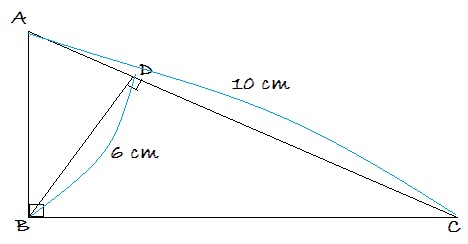# Area Games 2K16

Geometry Level 2

Find the area of a right triangle whose hypotenuse is 10 cm and altitude to its hypotenuse is 6 cm in sq cm.Note: The area of a triangle is equal to $\dfrac12 \times\text{ base } \times \text{ altitude }$.

×